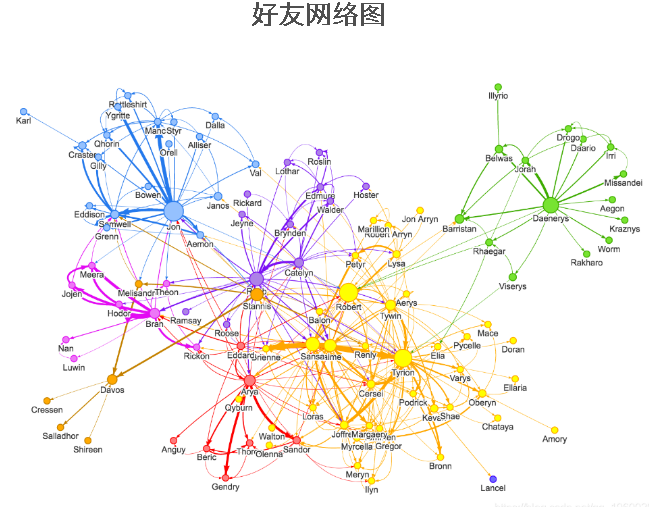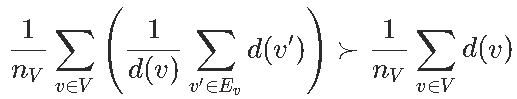# R语言网络分析友谊悖论案例

## 本文简要介绍一下网络分析，我想提供一些有关“友谊悖论”的R语言例证。友谊悖论指出

``````
library(networkD3)
simpleNetwork(data[,1:2]

​``````

###``````​
rbind(as.matrix(GoT[,1:2]),as.matrix(GoT[,2:1])
unique(M[,1]

​``````

``````as.character(M[which(M[,1]==x),2]
Vectorize(function(x) length(friends(x)

​``````

`````` (Vectorize(function(x) length(friends(x)))(friends(y
Vectorize(function(x) mean(friends_of_friends(x
​``````

``````
lines(density(Nb),col="red",
lines(density(Nb2),col="blue",

​``````

# python对网络图networkx进行社区检测和彩色绘图

``````​
mean(Nb)
 6.579439
mean(Nb2)
 13.94243

​``````

Kaizong Ye拓端研究室（TRL）的研究员。

​非常感谢您阅读本文，如需帮助请联系我们！QQ在线咨询

15121130882

0571-63341498

## 关注有关新文章的微信公众号

This will close in 0 seconds